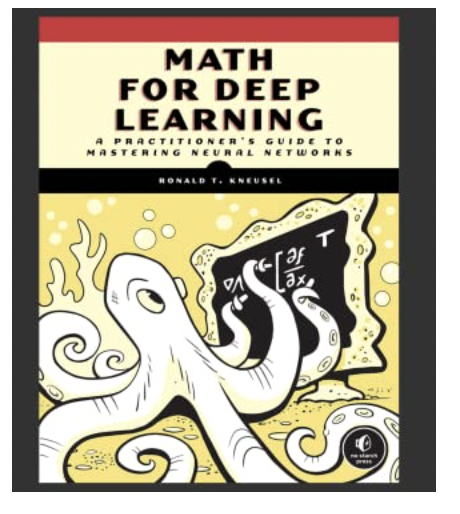# Outline

0 Setup

1 Probability

2 More Probability

3 Statistic

4 Linear Algebra

5 Differential Calculus

6 Matrix Calculus Data Flow in Neural Network

7 Data Flow in Neural Network

8 Backpropagation

## 1. Why do I need this work?

To lay groundwork for understanding the mathematical groundwork for Deep Learning.

Mostly all are creative ways to describe problems and representation.

## 2. Why should I care about Math?

Well -- you could speak another language, by investing yourself to speaking a new language, Mathematics.

One would establish formal jargon to speak Deep Learning.

Would you want to represent problems in n-dimensions?

Would you want to solve problems in high-dimensional space?

## 3. How much time would this take?

A week.

Reach out to me for notes, summary.

"The World is non-linear"